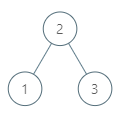# Leetcode - Inorder Successor in BST

violet posted @ Mar 24, 2020 10:28:50 AM in 算法 with tags Algorithm Golang tree , 52 阅读

https://leetcode.com/problems/inorder-successor-in-bst/

Given a binary search tree and a node in it, find the in-order successor of that node in the BST.

The successor of a node `p` is the node with the smallest key greater than `p.val`.

Example 1:```Input: root = [2,1,3], p = 1
Output: 2
Explanation: 1's in-order successor node is 2. Note that both p and the return value is of TreeNode type.
```

```/**
* Definition for a binary tree node.
* type TreeNode struct {
*     Val int
*     Left *TreeNode
*     Right *TreeNode
* }
*/
func inorderSuccessor(root *TreeNode, target *TreeNode) *TreeNode {
if root == nil {
return nil
}
stack := []*TreeNode{}
node := root
find := false
for node != nil || len(stack) != 0 {
for node != nil {
stack = append(stack, node)
node = node.Left
}
if len(stack) != 0 {
node = stack[len(stack)-1]
if find {
return node
}
if node == target {
find = true
}
node = node.Right
stack = stack[:len(stack)-1]

}
}
return nil
}```(输入验证码)
or Ctrl+Enter